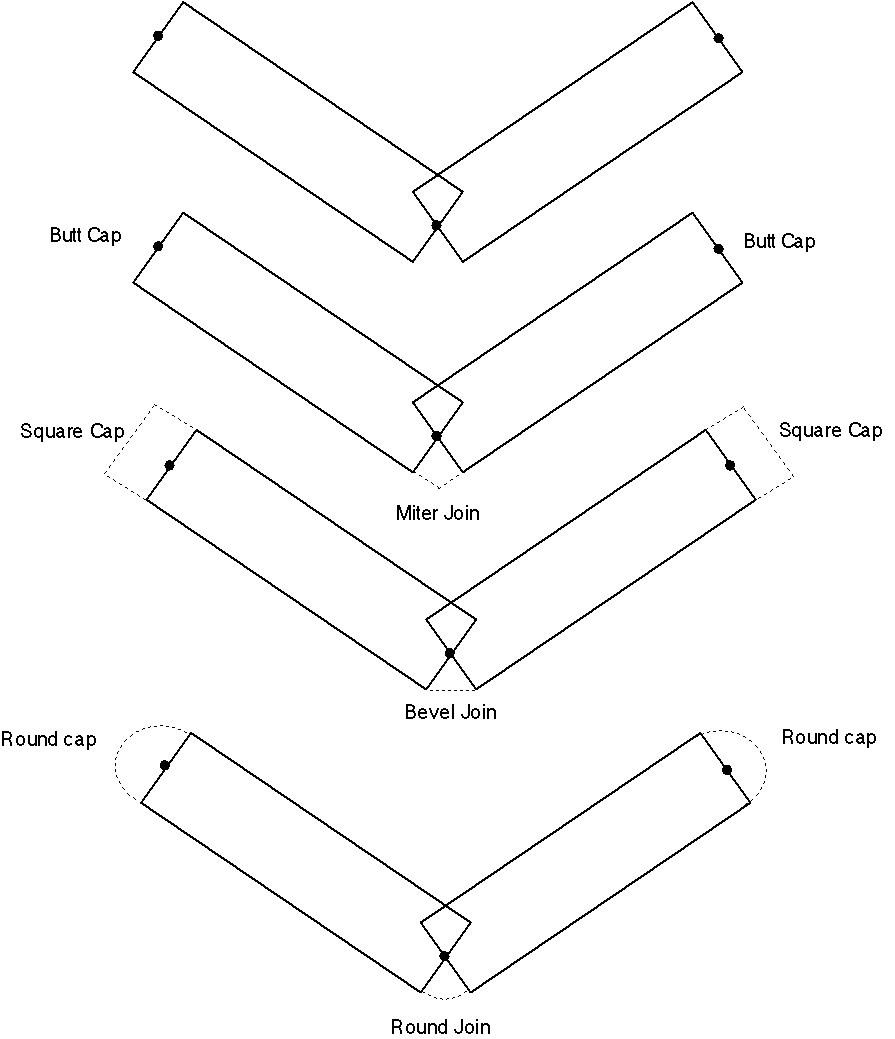Set Line Cap

Purpose

The Set Line Cap function specifies how the ends of thick (greater than one screen pixel) lines are rendered

Syntax

 Opcode format Opcode Operand Format Comments Extended ASCII (LineCap ) Defines how subsequent lines should be rendered.

Cap ASCII text string, describes how ends of thick lines should be rendered

Details

The Set Line Cap opcode specifies how the ends of subsequent thickened lines are rendered. The attribute applies to lines, polylines, circular arcs, and elliptical arcs. The meaning of the various line end-caps and joins are shown in figure 1.Figure 1. Caps and joins

The possible values for Cap include the following:

butt A butt line end-cap where the line terminates exactly at its endpoint

square A square line end-cap where the line is extended past its endpoint by an amount equal to half the line's weight

round A round line end-cap where the line is extended past its endpoint by a semicircle with a radius equal to half the line's weight

Notes

How the "line cap" attribute is applied to patterned lines is system dependent. Some DWF reading applications can apply the cap attributes to each portion of the line. For example, each dash and dashed line might have rounded end caps. If exact line patterns that synchronize with other geometric primitives in the drawing are required, then the DWF writing application should explicitly draw the individual dashes and dots required as a series of Draw Line calls.

Default

The default line cap is a butt end and is equivalent to

(LineCap butt)

Implementation in WHIP! Versions

 Toolkit Function TBD Partial Full Revised in Set Line Cap/Join/Pattern/Weight - 1.03 - -